### Practice Set 5.1 Co-ordinate Geometry Class 10th Mathematics Part 2 MHB Solution

Practice Set 5.1

1. Find the distance between each of the following pairs of points. (1) A(2, 3), B(4, 1)…
2. Determine whether the points are collinear. (1) A(1, -3), B(2, -5), C(-4, 7) (2) L(-2,…
3. Find the point on the X-axis which is equidistant from A(-3, 4) and B(1, -4).…
4. Verify that points P(-2, 2), Q(2, 2) and R(2, 7) are vertices of a right angled…
5. Show that points P(2, -2), Q(7, 3), R(11, -1) and S (6, -6) are vertices of a…
6. Show that points A(-4, -7), B(-1, 2), C(8, 5) and D(5, -4) are vertices of a rhombus…
7. Find x if distance between points L(x, 7) and M(1, 15) is 10.
8. Show that the points A(1, 2), B(1, 6), C(1 + 2 √3, 4) are vertices of an equilateral…

###### Practice Set 5.1

Question 1.

Find the distance between each of the following pairs of points.
(1) A(2, 3), B(4, 1)

(2) P(-5, 7), Q(-1, 3)

(3) R(0, -3), S(0, 5/2)

(4) L(5, -8), M(-7, -3)

(5) T(-3, 6), R(9, -10)

(6)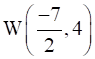, X(11, 4)

The distance between points A(x1, y1) and B(x2, y2) is given by,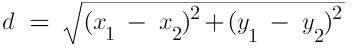1. Given Points: A(2, 3) and B(4, 1)
we can see that,
x1 = 2
x2 = 4
y= 3
y2 = 1
Putting the values in the distance formula we get,
d =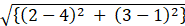⇒ d =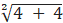⇒ d = √8

2. Given Points: P(-5, 7) and Q(-1, 3)
we can see that,
x1 = -5
x2 = -1
y1 = 7
y2 = 3
Putting these values in distance formula we get,

d =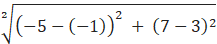d = √32

3. Given Points: R(0, -3), S(0, 5/2)
we can see that,
x1 = 0
x2 = 0
y1 = -3
y2 = 5/2

On putting these values in distance formula we get,

d =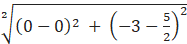d =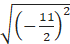d =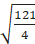4. Given Points: L(5, -8), M(-7, -3)

we can see that,
x1 = 5
x2 = -7
y1 = -8
y2 = -3

On putting these values in distance formula we get,

d =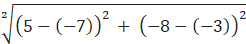d =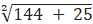d = √169 = 13

5. Given Points: T(-3, 6), R(9, -10)

we can see that,
x1 = -3
x2 = 9
y1 = 6
y2 = -10

On putting these values in distance formula we get,

d =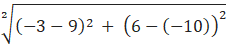d =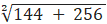d = 20

6. Given Points: W(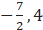), X(11, 4)

we can see that,
x1 = -7/2
x2 = 11
y1 = 4
y2 = 4

On putting these values in distance formula we get,

d =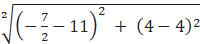d =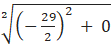d =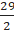Question 2.

Determine whether the points are collinear.

(1) A(1, -3), B(2, -5), C(-4, 7)

(2) L(-2, 3), M(1, -3), N(5, 4)

(3) R(0, 3), D(2, 1), S(3, -1)

(4) P(-2, 3), Q(1, 2), R(4, 1)

If Three points (a,b), (c,d), (e,f) are collinear then the area formed by the triangle by the three points is zero.

Area of a triangle =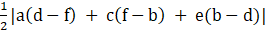...(1)

1.
(a,b) = (1,-3)

(c,d) = (2,-5)

(e,f) = (-4,7)

Area =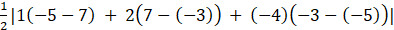Area =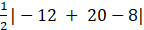= 0

Hence the points are collinear.

2.
(a,b) = (-2,3)

(c,d) = (1,-3)

(e,f) = (5,4)

Area =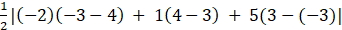Area =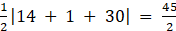Hence the points are not collinear.

3.
(a,b) = (0,3)

(c,d) = (2,1)

(e,f) = (3,-1)

Area =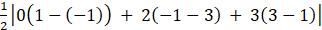Area =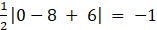Hence the points are non collinear.

4.
(a,b) = (-2,3)

(c,d) = (1,2)

(e,f) = (4,1)

Area =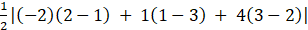Area =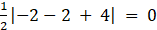Hence the points are collinear.

Question 3.

Find the point on the X-axis which is equidistant from A(-3, 4) and B(1, -4).

A point in the x = axis is of the form (a,0)

Distance d between two points(a,b) and (c,d)is given by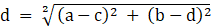Distance between (-3,4) and (a,0) =

D =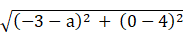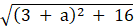Distance between (1,-4) and (a,0)

D =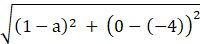D =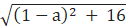As the two points are equidistant from the point (a.0)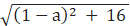=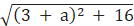Squaring both sides, we get

(1-a)2 + 16 = (3 + a)2 + 16

1 + a2 -2a = 9 + a2 + 6a

8a = -8

a = -1

Hence the point is (-1,0)

Question 4.

Verify that points P(-2, 2), Q(2, 2) and R(2, 7) are vertices of a right angled triangle.

In a right angles triangle ABC, right angled at B, according to the pythagoras theorem

AB2 + BC2 = AC2

According to the distance formula, the distance 'd' between two points (a,b) and (c,d) is given by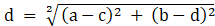.....(1)

For the given points Distance between P and Q is

PQ =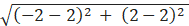=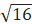QR =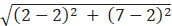=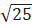PR =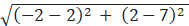=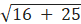=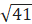PQ2 = 16

QR2 = 25

PR2 = 41

As PQ2 + QR2 = PR2

Hence the given points form a right angled triangle.

Question 5.

Show that points P(2, -2), Q(7, 3), R(11, -1) and S (6, -6) are vertices of a parallelogram.

In a parallelogram, opposite sides are equal and parallel.

According to the distance formula, the distance 'd' between two points (a,b) and (c,d) is given by.....(1)

For the given points, length PQ =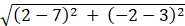PQ =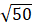Length QR =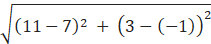QR =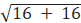=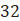Length RS =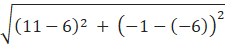RS =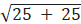=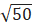Length SP =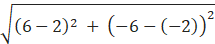SP ==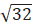As PQ = RS and QR = SP

Checking for slopes

Slope of a line between two points (a,b) and (c,d) is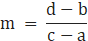Slope PQ =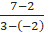= 1

Slope QR =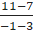=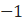Slope RS =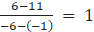Slope SP =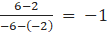As PQ = RS and their slope = 1

And

QR = SP and their slope = -1.

Hence the given points form a parallelogram.

Question 6.

Show that points A(-4, -7), B(-1, 2), C(8, 5) and D(5, -4) are vertices of a rhombus ABCD.

In a Rhombus the sides are equal and the diagonals bisect each other at 90°

According to the distance formula, the distance 'd' between two points (a,b) and (c,d) is given by.....(1)

Length AB =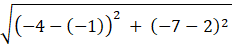=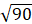Length BC =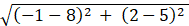=Length CD =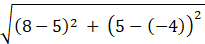=Length AD =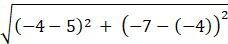=Slope of a line between two points (a,b) and (c,d) isSlope of Diagonal AC =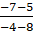= 1

Slope of diagonal BD =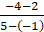= -1

Note: If the Product of slopes of two lines = -1 then they are perpendicular to each other.

As the product of slopes pf two diagonals = -1. Hence they're perpendicular to each other.

Hence The given points form a rhombus.

Question 7.

Find x if distance between points L(x, 7) and M(1, 15) is 10.

According to the distance formula, the distance 'd' between two points (a,b) and (c,d) is given by.....(1)

Distance between LM =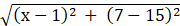= 10

Squaring both sides, we get

(x-1)2 + 64 = 100

(x-1)2 = 36

x-1 = ±6

Hence x = 7 or -5

Question 8.

Show that the points A(1, 2), B(1, 6), C(1 + 2 √3, 4) are vertices of an equilateral triangle.

For an equilateral triangle, all its sides are equal.

According to the distance formula, the distance 'd' between two points (a,b) and (c,d) is given by.....(1)

Length AB =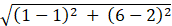=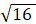= 4

Length BC =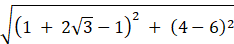=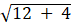= 4

Length AC =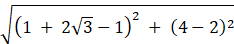== 4

Hence The given points form an equilateral triangle.

## PDF FILE TO YOUR EMAIL IMMEDIATELY PURCHASE NOTES & PAPER SOLUTION. @ Rs. 50/- each (GST extra)

SUBJECTS

HINDI ENTIRE PAPER SOLUTION

MARATHI PAPER SOLUTION
SSC MATHS I PAPER SOLUTION
SSC MATHS II PAPER SOLUTION
SSC SCIENCE I PAPER SOLUTION
SSC SCIENCE II PAPER SOLUTION
SSC ENGLISH PAPER SOLUTION
SSC & HSC ENGLISH WRITING SKILL
HSC ACCOUNTS NOTES
HSC OCM NOTES
HSC ECONOMICS NOTES
HSC SECRETARIAL PRACTICE NOTES

2019 Board Paper Solution

HSC ENGLISH SET A 2019 21st February, 2019

HSC ENGLISH SET B 2019 21st February, 2019

HSC ENGLISH SET C 2019 21st February, 2019

HSC ENGLISH SET D 2019 21st February, 2019

SECRETARIAL PRACTICE (S.P) 2019 25th February, 2019

HSC XII PHYSICS 2019 25th February, 2019

CHEMISTRY XII HSC SOLUTION 27th, February, 2019

OCM PAPER SOLUTION 2019 27th, February, 2019

HSC MATHS PAPER SOLUTION COMMERCE, 2nd March, 2019

HSC MATHS PAPER SOLUTION SCIENCE 2nd, March, 2019

SSC ENGLISH STD 10 5TH MARCH, 2019.

HSC XII ACCOUNTS 2019 6th March, 2019

HSC XII BIOLOGY 2019 6TH March, 2019

HSC XII ECONOMICS 9Th March 2019

SSC Maths I March 2019 Solution 10th Standard11th, March, 2019

SSC MATHS II MARCH 2019 SOLUTION 10TH STD.13th March, 2019

SSC SCIENCE I MARCH 2019 SOLUTION 10TH STD. 15th March, 2019.

SSC SCIENCE II MARCH 2019 SOLUTION 10TH STD. 18th March, 2019.

SSC SOCIAL SCIENCE I MARCH 2019 SOLUTION20th March, 2019

SSC SOCIAL SCIENCE II MARCH 2019 SOLUTION, 22nd March, 2019

XII CBSE - BOARD - MARCH - 2019 ENGLISH - QP + SOLUTIONS, 2nd March, 2019

HSC Maharashtra Board Papers 2020

(Std 12th English Medium)

HSC ECONOMICS MARCH 2020

HSC OCM MARCH 2020

HSC ACCOUNTS MARCH 2020

HSC S.P. MARCH 2020

HSC ENGLISH MARCH 2020

HSC HINDI MARCH 2020

HSC MARATHI MARCH 2020

HSC MATHS MARCH 2020

SSC Maharashtra Board Papers 2020

(Std 10th English Medium)

English MARCH 2020

HindI MARCH 2020

Hindi (Composite) MARCH 2020

Marathi MARCH 2020

Mathematics (Paper 1) MARCH 2020

Mathematics (Paper 2) MARCH 2020

Sanskrit MARCH 2020

Important-formula

THANKS